# FOIL

Here is a group of pictures that demonstrate what is meant by FOIL: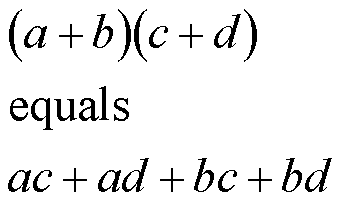First-OIL: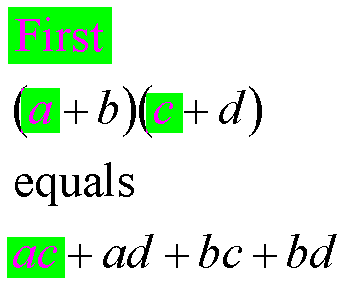Multiply the first terms of the binomial factors to get the first term of the result.

F-Outer-IL: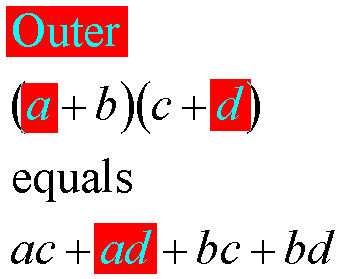Multiply the outer terms of the binomial factors to get the second term of the result.

FO-Inner-L: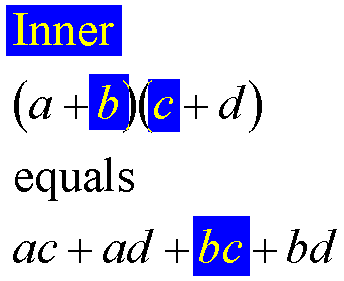Multiply the inner terms of the binomial factors to get the third term of the result.

FOI-Last: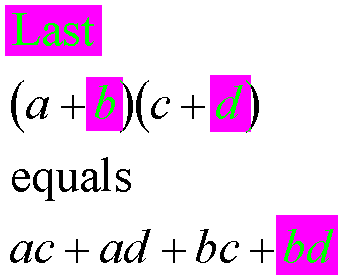Multiply the last terms of the binomial factors to get the last term of the result.

Custom Search